# Cesàro summation

There’s more than one way to sum an infinite series. Cesàro summation lets you compute the sum of series that don’t have a sum in the classical sense.

Suppose we have an infinite seriesThe nth partial sum of the series is given byThe classical sum of the series, if it exists, is defined to be the limit of its partial sums. That is,Cesàro summation takes a different approach. Instead of taking the limit of the partial sums, it takes the limit of the averages of the partial sums. To be specific, defineand define the Cesàro summation to be the limit of the Cn as n goes to infinity. If a series has a sum in the classical sense, it also has a sum in the Cesàro sense, and the limits are the same. But some series have a Cesàro sum that do not have a classical sum. Or maybe both limits exist but the intermediate steps of Cesàro summation are better behaved, as we’ll see in an example below.

If you express the Cn in terms of the original an terms you getIn other words, the nth Cesàro partial sum is a reweighting of the classical partial sums, with the weights changing as a function of n. Note that for fixed i, the fraction multiplying ai goes to 1 as n increases.

## Fejér summation and Gibbs phenomenon

Fejér summation
is Cesàro summation applied to Fourier series. The (ordinary) partial sums of a Fourier series give the best approximation to a function as measured by least squares norm. But the Cesàro partial sums may be qualitatively more like the function being approximated. We demonstrate this below with a square wave.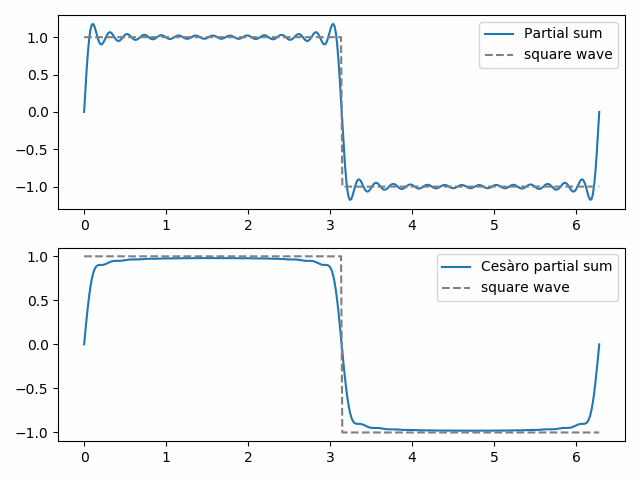The 30th ordinary partial sum shows the beginnings of Gibbs phenomenon, the “bat ears” at the top of the square wave and their mirror image at the bottom. The 30th Cesàro partial sum is smoother and eliminates Gibbs phenomena near the discontinuity in the square wave.

# Gibbs phenomenon

I realized recently that I’ve written about generalized Gibbs phenomenon, but I haven’t written about its original context of Fourier series. This post will rectify that.

The image below comes from a previous post illustrating Gibbs phenomenon for a Chebyshev approximation to a step function.Although Gibbs phenomena comes up in many different kinds of approximation, it was first observed in Fourier series, and not by Gibbs . This post will concentrate on Fourier series, and will give an example to correct some wrong conclusions one might draw about Gibbs phenomenon from the most commonly given examples.

The uniform limit of continuous function is continuous, and so the Fourier series of a function cannot converge uniformly where the function is discontinuous. But what does the Fourier series do near a discontinuity?

It’s easier to say what the Fourier series does exactly at a discontinuity. If a function is piecewise continuous, then the Fourier series at a jump discontinuity converges to the average of the limits from the left and from the right at that point.

What the Fourier series does on either side of the discontinuity is more interesting. You can see high-frequency oscillations on either side. The series will overshoot on the high side of the jump and undershoot on the low side of the jump.

The amount of overshoot and undershoot is proportional to the size of the gap, about 9% of the gap. The exact proportion, in the limit, is given by the Wilbraham-Gibbs constantGibbs phenomenon is usually demonstrated with examples that have a single discontinuity at the end of their period, such as a square wave or a saw tooth wave. But Gibbs phenomenon occurs at every discontinuity, wherever located, no matter how many there are.

The following example illustrates everything we’ve talked about above. We start with the function f plotted below on [-π, π] and imagine it extended periodically.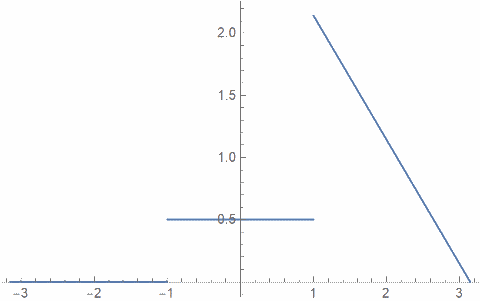1. It is continuous at the point where it repeats since it equals 0 at -π and π.
2. It has two discontinuities inside [-π, π].
3. One of the discontinuities is larger than the other.

The following plot shows the sum of the first 100 terms in the Fourier series for f plotted over [-2π, 2π].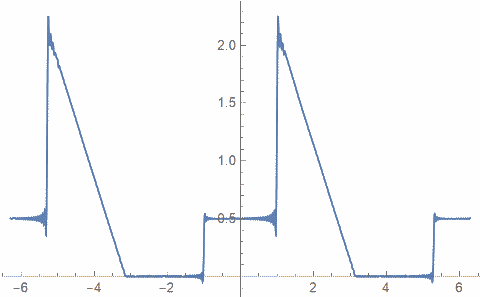1. There nothing remarkable about the series at -π and π.
2. You can see Gibbs phenomenon at the discontinuities of f.
3. The overshoot and undershoot are larger at the larger discontinuity.

Related to the first point above, note that the derivative of f is discontinuous at the period boundary. A discontinuity in the derivative does not cause Gibbs phenomena.

Here’s a close-up plot that shows the wiggling near the discontinuities.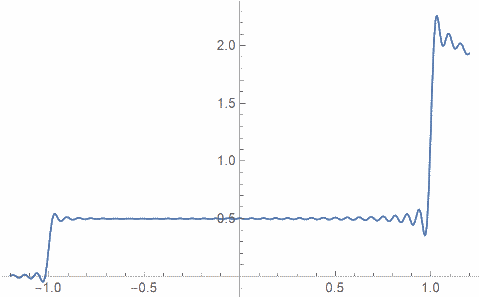## Gibbs phenomena for other series

 Henry Wilbraham first described what Josiah Gibbs discovered independently 50 years later, what we now call Gibbs phenomenon. This is an example of Stigler’s law of eponymy.

# Square wave, triangle wave, and rate of convergence

There are only a few examples of Fourier series that are relatively easy to compute by hand, and so these examples are used repeatedly in introductions to Fourier series. Any introduction is likely to include a square wave or a triangle wave .

By square wave we mean the function that is 1 on [0, 1/2] and -1 on [1/2, 1], extended to be periodic.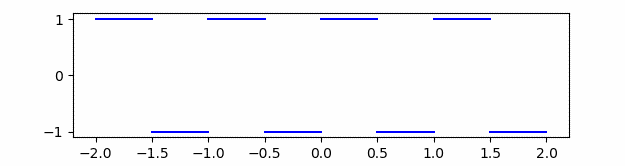Its nth Fourier (sine) coefficient is 4/nπ for odd n and 0 otherwise.

By triangle wave we mean 1 – |2x – 1|, also extended to be periodic.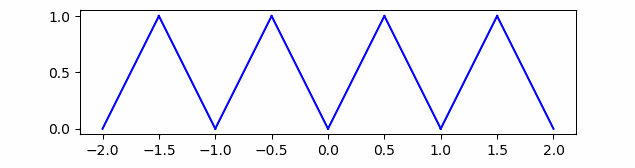Its nth Fourier (cosine) coefficient is -2/(nπ)² for odd n and 0 otherwise.

This implies the Fourier coefficients for the square wave are O(1/n) and the Fourier coefficients for the triangle wave are O(1/n²). (If you’re unfamiliar with big-O notation, see these notes.)

In general, the smoother a function is, the faster its Fourier coefficients approach zero. For example, we have the following two theorems.

1. If a function f has k continuous derivatives, then the Fourier coefficients are O(1/nk).
2. If the Fourier coefficients of f are O(1/nk+1+ε) for some ε > 0 then f has k continuous derivatives.

There is a gap between the two theorems so they’re not converses.

If a function is continuous but not differentiable, the Fourier coefficients cannot decay any faster than 1/n², so the Fourier coefficients for the triangle wave decay as fast as they can for a non-differentiable function.

## More post on rate of convergence

 The third canonical example is the saw tooth wave, the function f(x) = x copied repeatedly to make a periodic function. The function rises then falls off a cliff to start over. This discontinuity makes it like the square wave: its Fourier coefficients are O(1/n).

# Clipped sine waves

One source of distortion in electronic music is clipping. The highest and lowest portions of a wave form are truncated due to limitations of equipment. As the gain is increased, the sound doesn’t simply get louder but also becomes more distorted as more of the signal is clipped off.

## Clipping 0.2

For example, here is what a sine wave looks like when clipped 20%, i.e. cut off to be between -0.8 and 0.8.A simple sine wave has only one Fourier component, itself. But when we clip the sine wave, we move energy into higher frequency components. We can see that in the Fourier components below.You can show by symmetry that the even-numbered coefficients are exactly zero.

## Clipping 0.6

Here are the corresponding plots for 60% clipping, i.e. the absolute value of the signal is cut off to be 0.4. First the signaland then its Fourier components.Here are the first five sine waves with the amplitudes given by the Fourier coefficients.And here we see how the of the sines above do a pretty good job of reconstructing the original clipped sine. We’d need an infinite number of Fourier components to exactly reconstruct the original signal, but the first five components do most of the work.## Continuous range of clipping

Next let’s look at the ratio of the energy in the 3rd component to that of the 1st component as we continuously vary the amount of clipping.Now for the 5th harmonic. This one is interesting because it’s not strictly increasing but rather has a little bump before it starts increasing.Finally, here’s the ratio of the energy in all higher frequencies to the energy in the fundamental.# Building high frequencies out of low frequencies

If you have sines and cosines of some fundamental frequency, and you’re able to take products and sums, then you can construct sines and cosines at any multiple of the fundamental frequency.

Here’s a proof.Taking real parts gives us cos nθ in the first equation and the even terms of the sum in the last equation.

Taking imaginary parts gives us sin nθ in the first equation and the odd terms of the sum in the last equation.

# Sine series for a sine

The Fourier series of an odd function only has sine terms—all the cosine coefficients are zero—and so the Fourier series is a sine series.

What is the sine series for a sine function? If the frequency is an integer, then the sine series is just the function itself. For example, the sine series for sin(5x) is just sin(5x). But what if the frequency is not an integer?

For an odd function f on [-π, π] we havewhere the coefficients are given bySo if λ is not an integer, the sine series coefficients for sin(λx) are given byThe series converges slowly since the coefficients are O(1/n).

For example, here are the first 15 coefficients for the sine series for sin(1.6x).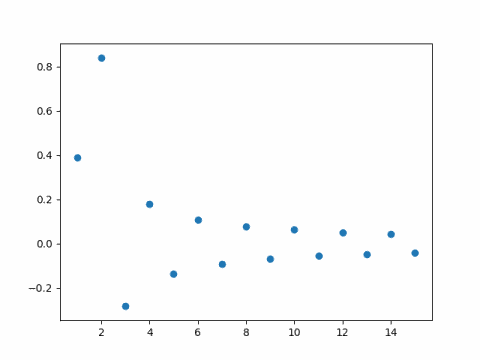And here is the corresponding plot for sin(2.9x).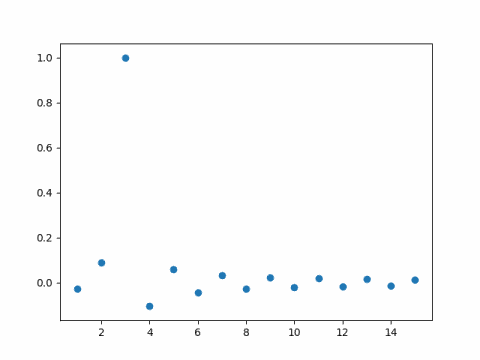As you might expect, the coefficient of sin(3x) is nearly 1, because 2.9 is nearly 3. What you might not expect is that the remaining coefficients are fairly large.

Update: After writing this post I wrote another on the rate of convergence for Fourier series. In general, the smoother the function, the faster the Fourier series converges and vice versa, with some fine print.

The sine function above is perfectly smooth, but it’s Fourier series converges slowly. How can that be? The Fourier series is defined for periodic functions. If k is not an integer and we force sin(kx) to be a function with period 2π, it is not continuous. When we extend sin(kx) to make it periodic, there’s a jump discontinuity at the ends of each period.

Look back atThis equation can’t hold everywhere. If k is not an integer and f(x) = sin(kx), then the right size is zero at π but the left is not. In fact we’ll see Gibbs phenomenon at π because of the discontinuity there.

# Exponential sum for the new year

Exponential sums can make intricate patterns. Last year I made a page that displays a different page each day, using the month, day, and year as parameters in the expression below. The images plot the partial sums of this sum.This was yesterday’s image.Today’s image is surprisingly plain if we use y = 18.This is in part because the least common multiple of 1, 1, and 18 is 18. The image could have no more than 18 vertices. In fact, it has only 6 vertices because the summand above has period 6.

But if we use y = 2018 we get something much more complex.The Exponential Sum of the Day page will use y = 18 this year. There will be a few simple images this year but there will also be lots of surprises.

# Moment generating functions and connections to other things

This post relates moment generating functions to the Laplace transform and to exponential generating functions. It also brings in connections to the z-transform and the Fourier transform.

Thanks to Brian Borchers who suggested the subject of this post in a comment on a previous post on transforms and convolutions.

## Moment generating functions

The moment generating function (MGF) of a random variable X is defined as the expected value of exp(tX). By the so-called rule of the unconscious statistician we havewhere fX is the probability density function of the random variable X. The function MX is called the moment generating function of X because it’s nth derivative, evaluated at 0, gives the nth moment of X, i.e. the expected value of Xn.

## Laplace transforms

If we flip the sign on t in the integral above, we have the two-sided Laplace transform of fX. That is, the moment generating function of X at t is the two-sided Laplace transform of fX at –t. If the density function is zero for negative values, then the two-sided Laplace transform reduces to the more common (one-sided) Laplace transform.

## Exponential generating functions

Since the derivatives of MX at zero are the moments of X, the power series for MX is the exponential generating function for the moments. We havewhere mn is the nth moment of X.

## Other generating functions

This terminology needs a little explanation since we’re using “generating function” two or three different ways. The “moment generating function” is the function defined above and only appears in probability. In combinatorics, the (ordinary) generating function of a sequence is the power series whose coefficient of xn is the nth term of the sequence. The exponential generating function is similar, except that each term is divided by n!. This is called the exponential generating series because it looks like the power series for the exponential function. Indeed, the exponential function is the exponential generating function for the sequence of all 1’s.

The equation above shows that MX is the exponential generating function for mn and the ordinary generating function for mn/n!.

If a random variable Y is defined on the integers, then the (ordinary) generating function for the sequence Prob(Yn) is called, naturally enough, the probability generating function for Y.

The z-transform of a sequence, common in electrical engineering, is the (ordinary) generating function of the sequence, but with x replaced with 1/z.

## Characteristic functions

The characteristic function of a random variable is a variation on the moment generating function. Rather than use the expected value of tX, it uses the expected value of itX. This means the characteristic function of a random variable is the Fourier transform of its density function.

Characteristic functions are easier to work with than moment generating functions. We haven’t talked about when moment generating functions exist, but it’s clear from the integral above that the right tail of the density has to go to zero faster than ex, which isn’t the case for fat-tailed distributions. That’s not a problem for the characteristic function because the Fourier transform exists for any density function. This is another example of how complex variables simplify problems.

# Shannon wavelet

The Shannon wavelet has an interesting plot:Given the complexity of the plot, the function definition is surprisingly simple:The Fourier transform is even simpler: it’s the indicator function of [-2π, -π] ∪ [π, 2π], i.e. the function that is 1 on the intervals [-2π, -π] and [π, 2π] but zero everywhere else.

The Shannon wavelet is orthogonal to integer translates of itself. This isn’t obvious in the time domain, but it’s easy to prove in the frequency domain using Parseval’s theorem.

Here’s a plot of the original wavelet and the wavelet shifted to the left by 3: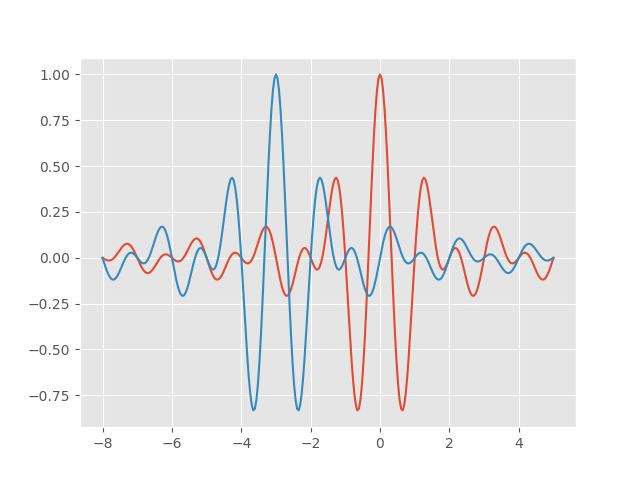And here’s a plot of the product of the two wavelets. It’s plausible that the areas above and below the x-axis cancel out each other, and indeed they do.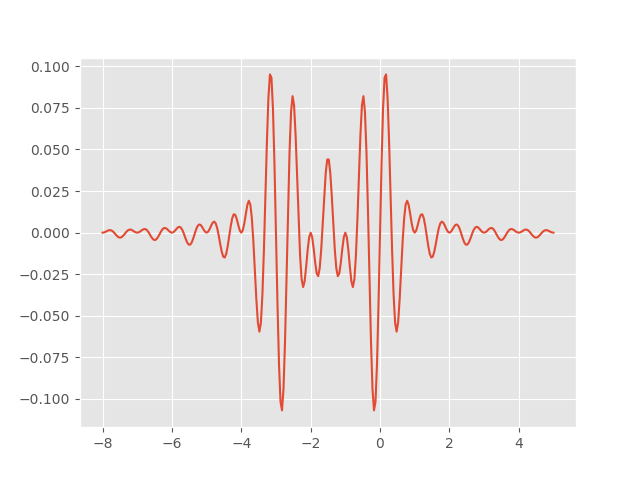Related post: Sinc and Jinc integrals

# Fourier-Bessel series and Gibbs phenomena

Fourier-Bessel series are analogous to Fourier series. And like Fourier series, they converge pointwise near a discontinuity with the same kind of overshoot and undershoot known as the Gibbs phenomenon.

## Fourier-Bessel series

Bessel functions come up naturally when working in polar coordinates, just as sines and cosines come up naturally when working in rectangular coordinates. You can think of Bessel functions as a sort of variation on sine waves. Or even more accurately, a variation on sinc functions, where sinc(z) = sin(z)/z. 

A Fourier series represents a function as a sum of sines and cosines of different frequencies. To make things a little simpler here, I’ll only consider Fourier sine series so I don’t have to repeatedly say “and cosine.”A Fourier-Bessel function does something similar. It represents a function as a sum of rescaled versions of a particular Bessel function. We’ll use the Bessel J0 here, but you could pick some other Jν.

Fourier series scale the sine and cosine functions by π times integers, i.e. sin(πz), sin(2πz), sin(3πz), etc. Fourier-Bessel series scale by the zeros of the Bessel function: J01z),  J02z),  J03z), etc. where λn are the zeros of J0. This is analogous to scaling sin(πz) by its roots: π, 2π, 3π, etc. So a Fourier-Bessel series for a function f looks likeThe coefficients cn for Fourier-Bessel series can be computed analogously to Fourier coefficients, but with a couple minor complications. First, the basis functions of a Fourier series are orthogonal over [0, 1] without any explicit weight, i.e. with weight 1. And second, the inner product of a basis function doesn’t depend on the frequency. In detail,Here δmn equals 1 if m = n and 0 otherwise.

Fourier-Bessel basis functions are orthogonal with a weight z, and the inner product of a basis function with itself depends on the frequency. In detailSo whereas the coefficients for a Fourier sine series are given bythe coefficients for a Fourier-Bessel series are given by## Gibbs phenomenon

Fourier and Fourier-Bessel series are examples of orthogonal series, and so by construction they converge in the norm given by their associated inner product. That means that if SN is the Nth partial sum of a Fourier seriesand the analogous statement for a Fourier-Bessel series isIn short, the series converge in a (weighted) L² norm. But how do the series converge pointwise? A lot of harmonic analysis is devoted to answering this question, what conditions on the function f guarantee what kind of behavior of the partial sums of the series expansion.

If we look at the Fourier series for a step function, the partial sums converge pointwise everywhere except at the step discontinuity. But the way they converge is interesting. You get a sort of “bat ear” phenomena where the partial sums overshoot the step function at the discontinuity. This is called the Gibbs phenomenon after Josiah Willard Gibbs who observed the effect in 1899. (Henry Wilbraham observed the same thing earlier, but Gibbs didn’t know that.)

The Gibbs phenomena is well known for Fourier series. It’s not as well known that the same phenomenon occurs for other orthogonal series, such as Fourier-Bessel series. I’ll give an example of Gibbs phenomenon for Fourier-Bessel series taken from  and give Python code to visualize it.

We take our function f(z) to be 1 on [0, 1/2] and 0 on (1/2, 1]. It works out that## Python code and plot

Here’s the plot with 100 terms. Notice how the partial sums overshoot the mark to the left of 1/2 and undershoot to the right of 1/2.Here’s the same plot with 1,000 terms.Here’s the Python code that produced the plot.

    import matplotlib.pyplot as plt
from scipy.special import j0, j1, jn_zeros
from scipy import linspace

N = 100 # number of terms in series

roots = jn_zeros(0, N)
coeff = [j1(r/2) / (r*j1(r)**2) for r in roots]
z = linspace(0, 1, 200)

def partial_sum(z):
return sum( coeff[i]*j0(roots[i]*z) for i in range(N) )

plt.plot(z, partial_sum(z))
plt.xlabel("z")
plt.ylabel("{}th partial sum".format(N))
plt.show()


## Footnotes

 To be precise, as z goes to infinityand so the Bessel functions are asymptotically proportional to sin(z – φ)/√z for some phase shift φ.

 The Gibbs’ phenomenon for Fourier-Bessel Series. Temple H. Fay and P. Kendrik Kloppers. International Journal of Mathematical Education in Science and Technology. 2003. vol. 323, no. 2, 199-217.